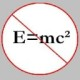# The Unapologetic Mathematician

## Hopf Algebras

One more piece of structure we need. We take a bialgebra$H$, and we add an “antipode”, which behaves sort of like an inverse operation. Then what we have is a Hopf algebra.

An antipode will be a linear map$S:H\rightarrow H$ on the underlying vector space. Here’s what we mean by saying that an antipode “behaves like an inverse”. In formulas, we write that:$\mu\circ(S\otimes1_H)\circ\Delta=\iota\circ\epsilon=\mu\circ(1_H\otimes S)\circ\Delta$

On either side, first we comultiply an algebra element to split it into two parts. Then we use$S$ on one or the other part before multiplying them back together. In the center, this is the same as first taking the counit to get a field element, and then multiplying that by the unit of the algebra.

By now it shouldn’t be a surprise that the group algebra$\mathbb{F}[G]$ is also a Hopf algebra. Specifically, we set$S(e_g)=e_{g^{-1}}$. Then we can check the “left inverse” law:\begin{aligned}\mu\left(\left[S\otimes1_H\right]\left(\Delta(e_g)\right)\right)=\mu\left(\left[S\otimes1_H\right](e_g\otimes e_g)\right)=\\\mu(e_{g^{-1}}\otimes e_g)=e_{g^{-1}g}=e_1=\iota(1)=\iota\left(\epsilon(e_g)\right)\end{aligned}

One thing that we should point out: this is not a group object in the category of vector spaces over$\mathbb{F}$. A group object needs the diagonal we get from the finite products on the target category. But in the category of vector spaces we pointedly do not use the categorical product as our monoidal structure. There is no “diagonal” for the tensor product.

Instead, we move to the category of coalgebras over$\mathbb{F}$. Now each coalgebra$C$ comes with its own comultiplication$\Delta:C\rightarrow C\otimes C$, which stands in for the diagonal. In the case of$\mathbb{F}[G]$ we’ve been considering, this comultiplication is clearly related to the diagonal on the underlying set of the group$G$. In fact, it’s not going too far to say that “linearizing” a set naturally brings along a coalgebra structure on top of the vector space structure we usually consider. But many coalgebras, bialgebras, and Hopf algebras are not such linearized sets.

In the category of coalgebras over$\mathbb{F}$, a Hopf algebra is a group object, so long as we use the comultiplications and counits that come with the coalgebras instead of the ones that come from the categorical product structure. Dually, we can characterize a Hopf algebra as a cogroup object in the category of algebras over$\mathbb{F}$, subject to a similar caveat. It is this cogroup structure that will be important moving forwards.

November 7, 2008 - Posted by | Algebra

## 9 Comments »

1. Thank you! This is a subject which I’ve had a hard time learning from books and journal articles. I’m driven to try because of the special cases known as “called quantum groups” and because of categories of representations as a motivation for Hopf algebras.

PlanetMath says, for instance:

“The category of commutative Hopf algebras is anti-equivalent to the category of affine group schemes. The prime spectrum of a commutative Hopf algebra is an affine group scheme of multiplicative units. And going in the opposite direction, the algebra of natural transformations from an affine group scheme to its affine 1-space is a commutative Hopf algebra, with coalgebra structure given by dualising the group structure of the affine group scheme. Further, a commutative Hopf algebra is a cogroup object in the category of commutative algebras.”

Polynomial functions on a Lie group looks like it should have PHysics applications that I never understood when presented to me by Physicists. Nothing person, my wife being a Physics professor, after all…Comment by Jonathan Vos Post | November 7, 2008 | Reply

2. Polynomial functions on a Lie group looks like it should have PHysics applications

Well, most Lie groups you might be thinking of are also algebraic groups, which is why polynomial functions would be apropos. The algebra of such functions will be another Hopf algebra — an algebro-geometric analogue of the group algebra I talk about above.Comment by John Armstrong | November 7, 2008 | Reply

3. So how does this count as mathematics for the interested outsider?

It’s interesting, but not for the outsider!

You have to use less technical language to appeal to the masses, who don’t know very much.Comment by notedscholar | November 8, 2008 | Reply

4. If the outsider starts at the beginning, they’d have a considerably easier time. Some things just don’t translate to “lay language”.Comment by Blake Stacey | November 8, 2008 | Reply

5. (Hmmm, the beginning was actually before the post to which I linked, which was more like the beginning of the middle.)Comment by Blake Stacey | November 8, 2008 | Reply

6. Try following back the links I give that refer to earlier posts. And then follow those links, and so on until you find illumination.

It’s postmodern satoriComment by John Armstrong | November 8, 2008 | Reply

7. […] if we can swap the outputs from the comultiplication. That is, if . Similarly, bialgebras and Hopf algebras can be […]

Pingback by Cocommutativity « The Unapologetic Mathematician | November 19, 2008 | Reply

8. […] let’s say we have a group . This gives us a cocommutative Hopf algebra. Thus the category of representations of is monoidal — symmetric, even — and has […]

Pingback by The Category of Representations of a Group « The Unapologetic Mathematician | November 21, 2008 | Reply

9. Pingback by Useful links on Hopf Algebras | gavinsmathnotes | November 7, 2015 | Reply